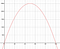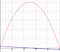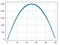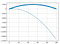## Machine Learning is Applied Calculus

• Understand the use of the derivative and gradient to find the “direction of maximum increase.”

# Reviewing Optimization Problems and Calculus

You have purchased 200 meters of wire-mesh fencing. You want to use this fence to create a rectangular pasture for your flock of sheep. How can you determine the length and width that maximizes the area inside of your pasture?

`area = length * width(2*length) + (2*width) = 200`
`2*width = 200–2*lengthwidth = [200–2*length] / 2width = 100 — length`
`A(length) = length * [100 — length]A(length) = 100*length — length²`
`A’(length) = 100–2*length`
`A’(length) = 0A’(length) = 100–2*length100–2*length = 0-2*length = -100length = 50`
`A(length) = 100*length — length²a(49) = 4900–2401 = 2499a(50) = 5000–2500 = 2500a(51) = 5100–2601 = 2499`A global maximum appears at length=50 — just like the derivative told us.

# Gradient Descent — Iterative Guesswork

So, what does all this have to do with gradient descent? Gradient descent is brutish, like the imprecise guesswork we just did. It’s not an elegant formula, it’s a messy series of ever so slightly better guesses. Here’s how it would work for our fencing problem:

1. Compute the value of the derivative at that point.
3. Repeat until you guess right.
`A’(57) = 100–2*(57)A’(57) = -14`
`57–3 = 54A(54) = 5400 — (54²)A(54) = 5400–2916A(54) = 2484`
`A’(length) = 100–2*54 = 100–108 = -8`
`A(52) = 5200–52²A(52) = 5200–2704A(52) = 2496`Notice the derivative (blue) — it’s negative to the right of the maximum, and positive to the left of the maximum. In this case it’s easy to follow the derivative directly the the max.

# Gradient Descent For Function Finding

In the prior example we had a curve and we used gradient descent to find an optimal value along that curve. In machine learning we have a collection of data points and we want to create a curve that satisfactorily fits those data-points. Let’s take the fencing problem we just examined and make it more like the problems we tend to solve with machine learning:It looks like there is a pattern in this data… can we use machine learning to define the pattern?
`F(x) = ax² + bx`
`L(x) = | F(x) — y |`
`L(x) = | -2x² + 30x + 0 — y |`Oops, we start pretty close to our scatterplot points at x=0 but by the time x=100 we are way off. The absolute error of a single datapoint the length of the vertical line that connects a point in the scatter plot to the point below it on the line. The mean absolute error is the average length of all those vertical lines.
`L(a, b) = ax² + bx — y; where x and y are treated as constants.`
`L(a, b) = 1/m * SUM(| F(a, b) — yi | )L(a, b) = (1/m) * SUM( | axi² + bxi — yi | )`
`d/dx |x| =  1 if x is positive andd/dx |x| = -1 if x is negative.`
`L(a, b) = (1/m) * SUM( | axi² + bxi — yi | )L’a(a, b) = (1 / m) * SUM( 1 * xi² );  if F(xi) > yiL’a(a, b) = (1 / m) * SUM( -1 * xi² ); if F(xi) < yiL’b(a, b) = (1 / m) * SUM( 1 * x );  if F(xi) > yL’b(a, b) = (1 / m) * SUM( -1 * x ); if F(xi) < yi`
`import numpy as npx = np.arange(0,100,1)y_true = [100*p — p*p for p in x] # b = 100 a = 1, the ground truthy_est = [30*p — 2*p*p for p in x] # b = 30 a = 2, our guesserror = sum([ye — yt for ye, yt in zip(y_est, y_true)])mean_error = error / 100# error = -674850# mean_error = -67485`

Written by